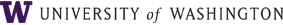# Instructor Class Description

Time Schedule:

Dana Dabiri
M E 503
Seattle Campus

### Continuum Mechanics

Reviews concepts of motion, stress, energy for a general continuum; conservation of mass, momentum, and energy; and the second law; constitutive equations for linear/nonlinear elastic, viscous/inviscid fluids, and general materials; and examples/solutions for solid/fluid materials. Offered: jointly with A A 503; A.

Class description

Continuum mechanics is a branch of mechanics that deals with the kinematics and mechanical behavior of materials modeled as a continuum, i.e., solids and fluids (e.g., liquids and gases). A continuum concept assumes that the substance of the body is distributed throughout — and completely fills — the space it occupies. The continuum concept ignores the fact that matter is made of atoms and that it has some sort of heterogeneous microstructure, but uses the approximation of physical quantities such as energy and momentum in the infinitesimal limit. Partial differential equations can thus be employed in solving problems in continuum mechanics. Some of these differential equations are specific to the materials being investigated and are called constitutive equations, while others capture fundamental physical laws, such as the conservation of mass (the continuity equation), the conservation of momentum (the equations of motion and equilibrium), and the conservation of energy (the first law of thermodynamics). Continuum mechanics deals with physical quantities of solids and fluids and those are independent of any particular coordinate system in which they are observed. These physical quantities are then represented by tensors, which are mathematical objects that are independent of coordinate system. These tensors can be expressed in coordinate systems for computational convenience. This course discusses those concepts of motion, stress, energy for a general continuum; conservation of mass, momentum, and energy; and the second law; constitutive equations for linear/nonlinear elastic, viscous/inviscid fluids, and general materials; and gives examples and solutions for solid and fluid materials.

Student learning goals

General method of instruction

Recommended preparation

Class assignments and grading

The information above is intended to be helpful in choosing courses. Because the instructor may further develop his/her plans for this course, its characteristics are subject to change without notice. In most cases, the official course syllabus will be distributed on the first day of class.
Last Update by Dana Dabiri
Date: 09/29/2010